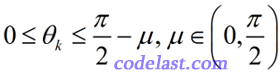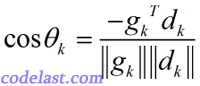# [原创]使用一维搜索(line search)的算法的收敛性

【1】搜索方向条件

${d_k}$ 与负梯度 $- {g_k}$ 的夹角 ${\theta _k}$ 来衡量搜索方向。我们先给出结论： ${\theta _k}$ 应满足：$f({x_k} + \alpha {d_k}) = f({x_k}) + \alpha {g_k}^T{d_k} + o(\alpha )$

【2】两个关于收敛性的重要理论

（1）存在某个有限的 $k$ ，使得 ${g_k} = 0$
（2） $f({x_k}) \to - \infty$
（3） ${g_k} \to 0$

（3）中的 ${g_k} \to 0$ 又是个什么概念呢？大家可以想像一下二维平面上的寻优过程，一个图像类似于抛物线的函数，当搜索点逐渐向极小值点逼近时，其梯度 ${g_k}$ 正是趋于0的。

（1）存在某个有限的 $k$ ，使得 ${g_k} = 0$
（2） $f({x_k}) \to - \infty$
（3） ${g_k} \to 0$

➤➤ 版权声明 ➤➤